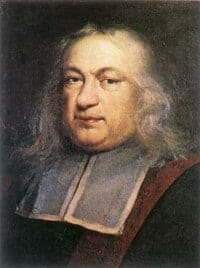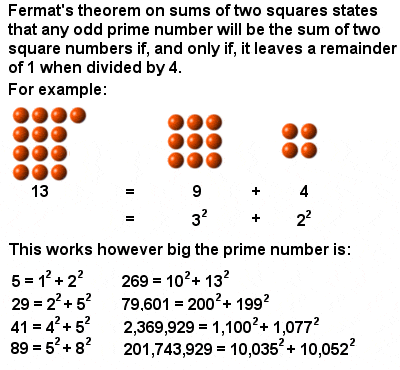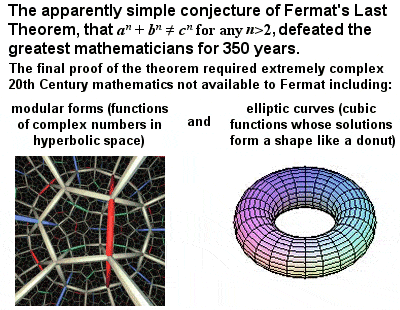# PIERRE DE FERMAT MATHEMATICIAN

## BiographyPierre de Fermat (1601-1665)

Another Frenchman of the 17th Century, Pierre de Fermat, effectively invented modern number theory virtually single-handedly, despite being a small-town amateur mathematician. Stimulated and inspired by the “Arithmetica” of the Hellenistic mathematician Diophantus, he went on to discover several new patterns in numbers which had defeated mathematicians for centuries, and throughout his life he devised a wide range of conjectures and theorems. He is also given credit for early developments that led to modern calculus, and for early progress in probability theory.

Although he showed an early interest in mathematics, he went on study law at Orléans and received the title of councillor at the High Court of Judicature in Toulouse in 1631, which he held for the rest of his life. He was fluent in Latin, Greek, Italian and Spanish, and was praised for his written verse in several languages, and eagerly sought for advice on the emendation of Greek texts.

Fermat’s mathematical work was communicated mainly in letters to friends, often with little or no proof of his theorems. Although he himself claimed to have proved all his arithmetic theorems, few records of his proofs have survived, and many mathematicians have doubted some of his claims, especially given the difficulty of some of the problems and the limited mathematical tools available to Fermat.

## The Two Square TheoremFermat’s Theorem on Sums of Two Squares

One example of his many theorems is the Two Square Theorem, which shows that any prime number which, when divided by 4, leaves a remainder of 1 (i.e. can be written in the form 4n + 1), can always be re-written as the sum of two square numbers (see image at right for examples).

His so-called Little Theorem is often used in the testing of large prime numbers, and is the basis of the codes which protect our credit cards in Internet transactions today. In simple (sic) terms, it says that if we have two numbers a and p, where p is a prime number and not a factor of a, then a multiplied by itself p-1 times and then divided by p, will always leave a remainder of 1. In mathematical terms, this is written: ap-1 = 1(mod p). For example, if a = 7 and p = 3, then 72 ÷ 3 should leave a remainder of 1, and 49 ÷ 3 does in fact leave a remainder of 1.

## Fermat numbers

Fermat identified a subset of numbers, now known as Fermat numbers, which are of the form of one less than 2 to the power of a power of 2, or, written mathematically, 22n + 1. The first five such numbers are: 21 + 1 = 3; 22 + 1 = 5; 24 + 1 = 17; 28 + 1 = 257; and 216 + 1 = 65,537. Interestingly, these are all prime numbers (and are known as Fermat primes), but all the higher Fermat numbers which have been painstakingly identified over the years are NOT prime numbers, which just goes to to show the value of inductive proof in mathematics.

## Last TheoremFermat’s Last Theorem

Fermat’s pièce de résistance, though, was his famous Last Theorem, a conjecture left unproven at his death, and which puzzled mathematicians for over 350 years. The theorem, originally described in a scribbled note in the margin of his copy of Diophantus‘ “Arithmetica”, states that no three positive integers a, b and c can satisfy the equation an + bn = cn for any integer value of n greater than two (i.e. squared). This seemingly simple conjecture has proved to be one of the world’s hardest mathematical problems to prove.

There are clearly many solutions – indeed, an infinite number – when n = 2 (namely, all the Pythagorean triples), but no solution could be found for cubes or higher powers. Tantalizingly, Fermat himself claimed to have a proof, but wrote that “this margin is too small to contain it”. As far as we know from the papers which have come down to us, however, Fermat only managed to partially prove the theorem for the special case of n = 4, as did several other mathematicians who applied themselves to it (and indeed as had earlier mathematicians dating back to Fibonacci, albeit not with the same intent).

Over the centuries, several mathematical and scientific academies offered substantial prizes for a proof of the theorem, and to some extent it single-handedly stimulated the development of algebraic number theory in the 19th and 20th Centuries. It was finally proved for ALL numbers only in 1995 (a proof usually attributed to British mathematician Andrew Wiles, although in reality it was a joint effort of several steps involving many mathematicians over several years). The final proof made use of complex modern mathematics, such as the modularity theorem for semi-stable elliptic curves, Galois representations and Ribet’s epsilon theorem, all of which were unavailable in Fermat’s time, so it seems clear that Fermat’s claim to have solved his last theorem was almost certainly an exaggeration (or at least a misunderstanding).

In addition to his work in number theory, Fermat anticipated the development of calculus to some extent, and his work in this field was invaluable later to Newton and Leibniz. While investigating a technique for finding the centres of gravity of various plane and solid figures, he developed a method for determining maxima, minima and tangents to various curves that was essentially equivalent to differentiation. Also, using an ingenious trick, he was able to reduce the integral of general power functions to the sums of geometric series.

Fermat’s correspondence with his friend Pascal also helped mathematicians grasp a very important concept in basic probability which, although perhaps intuitive to us now, was revolutionary in 1654, namely the idea of equally probable outcomes and expected values.